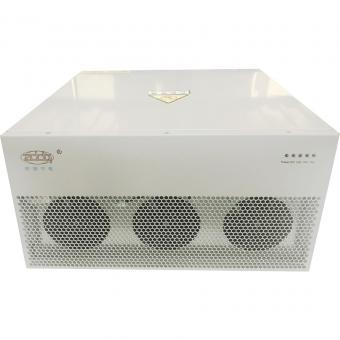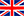English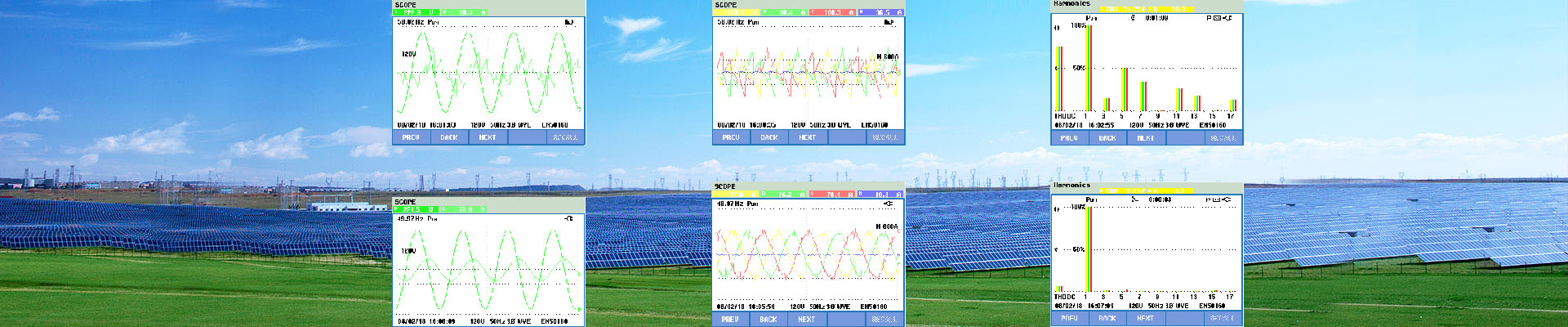###### Sizing Active Harmonic Filter from Power Analyser Data
Jun 16, 2018

The Graph below shows Harmonic Current Distortion in the form of %THDi. This is hovering between 20 and 25%. Ideally, it should be less than 10% and preferably less than 8% THDi.

The highest value of average total harmonic distortion (%THDi) across three phases was calculated from raw data. This value is 24.19%THDi and was recorded at time shown.

At the same time, average line current (Amps) across three phases was 516.83A.

Analysis of Findings
From data above, Highest Average %THDi = 24.19%

At the same time, Average I RMS across three phases was 516.83A.

Taking X to be = fundamental frequency current

I RMS = √(12 + 0.24192) * x = 516.83A
1.02884 * x = 516.83
x = 516.83 / 1.02884 = 502.34A

Calculate harmonic current

I RMS = √(502.342 + Harmonic Current2) = 516.83A
502.342 + Harmonic Current2 = 267113
Harmonic Current2 = 267113 – 252345 = 14767.8A
Thus Harmonic current = 121.52A

If %THDi = 8% (An appropriate value to satisfy electrical supply utilities)
I RMS = √(502.342 + (0.08*502.34)2)
= √(252345 + 1615)
=503.94A

I RMS = √(502.342 + Harmonic Current2) = 503.94A
502.342 + Harmonic Current2 = 253955.5
Harmonic Current2 = 253955.5 – 252345.5 = 1610A
Thus Harmonic current = 40.125A

Thus– To reduce % THDi at Incomer from 24.91% to 8% requires 81.4A of harmonic filtering (121.52-40.125). Active Harmonic filters come in sizes of 60A, 120A, 200A and 300A. To reduce %THDi to a level of 8% would require a 120A filter.

Check performance when filter installed.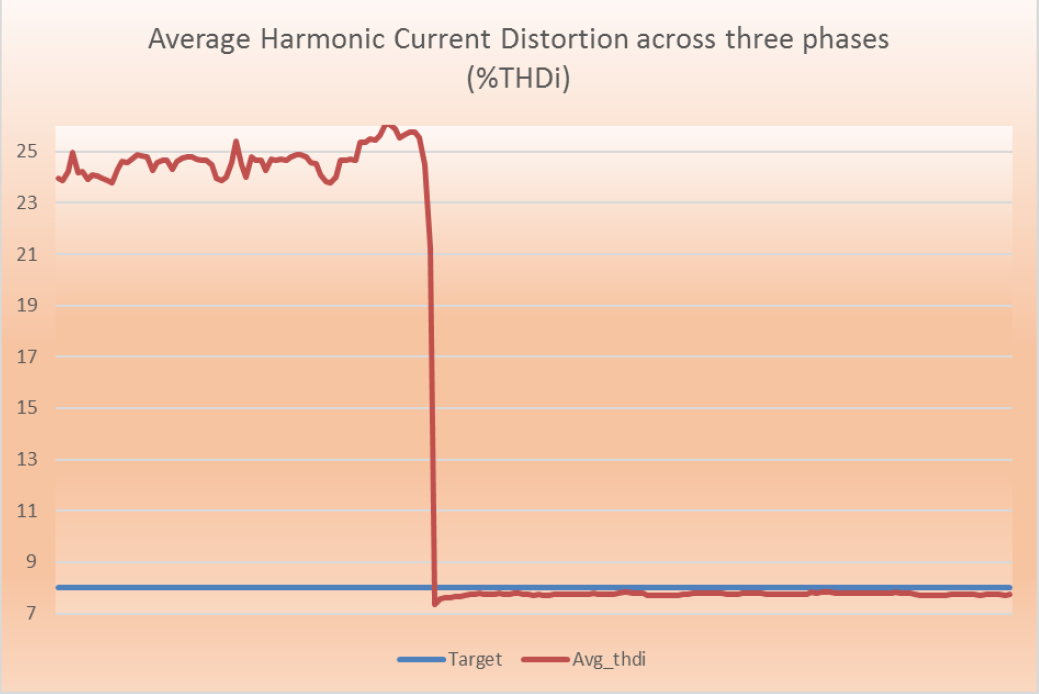Hot Products
•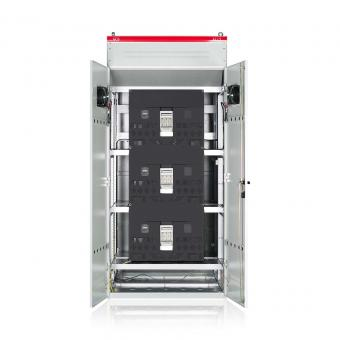#### Capacitor banks and harmonic filters. Low voltage

•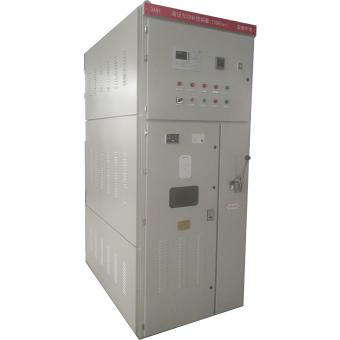#### 3.3KV Local reactive power compensation for motors,Arc Furnace

•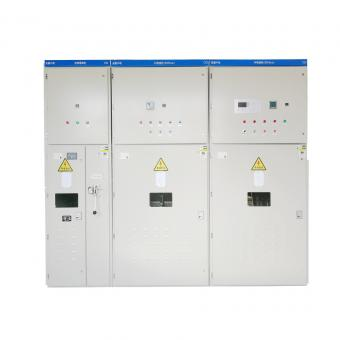#### Medium voltage Automatic Capacitor banks with harmonic filters

•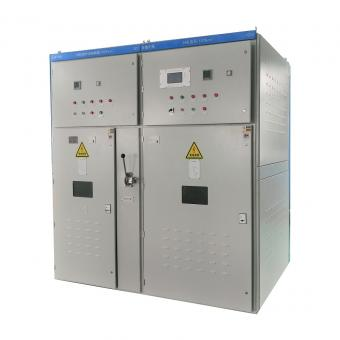#### High voltage Automatic Power Factor improvement plant for Motors VFDs

•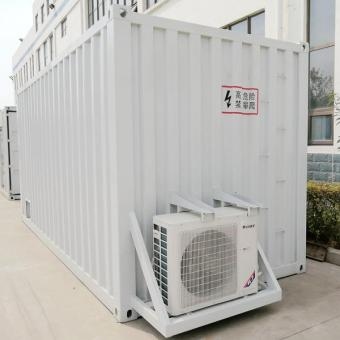#### High voltage static var generator HV SVG Statcom outdoor container

•#### Medium voltage outdoor Static Synchronous Compensator(STATCOM)

•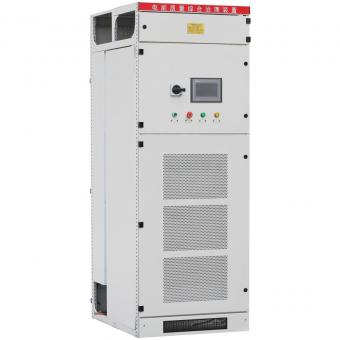•